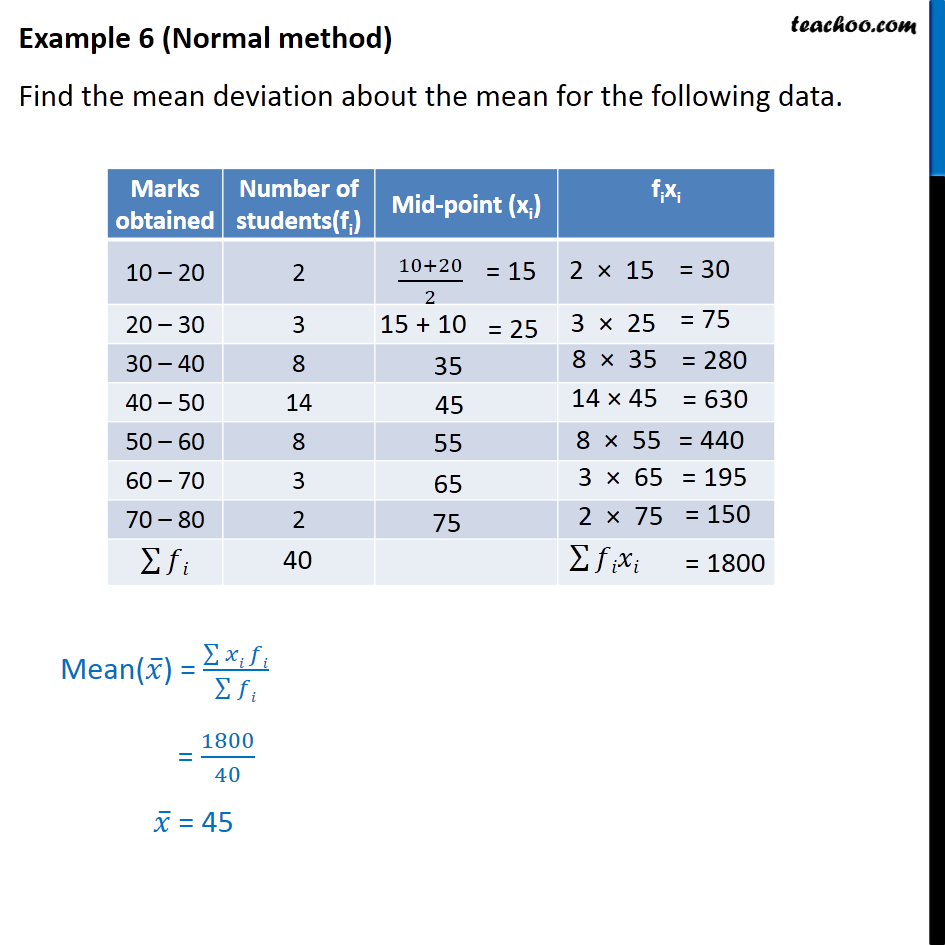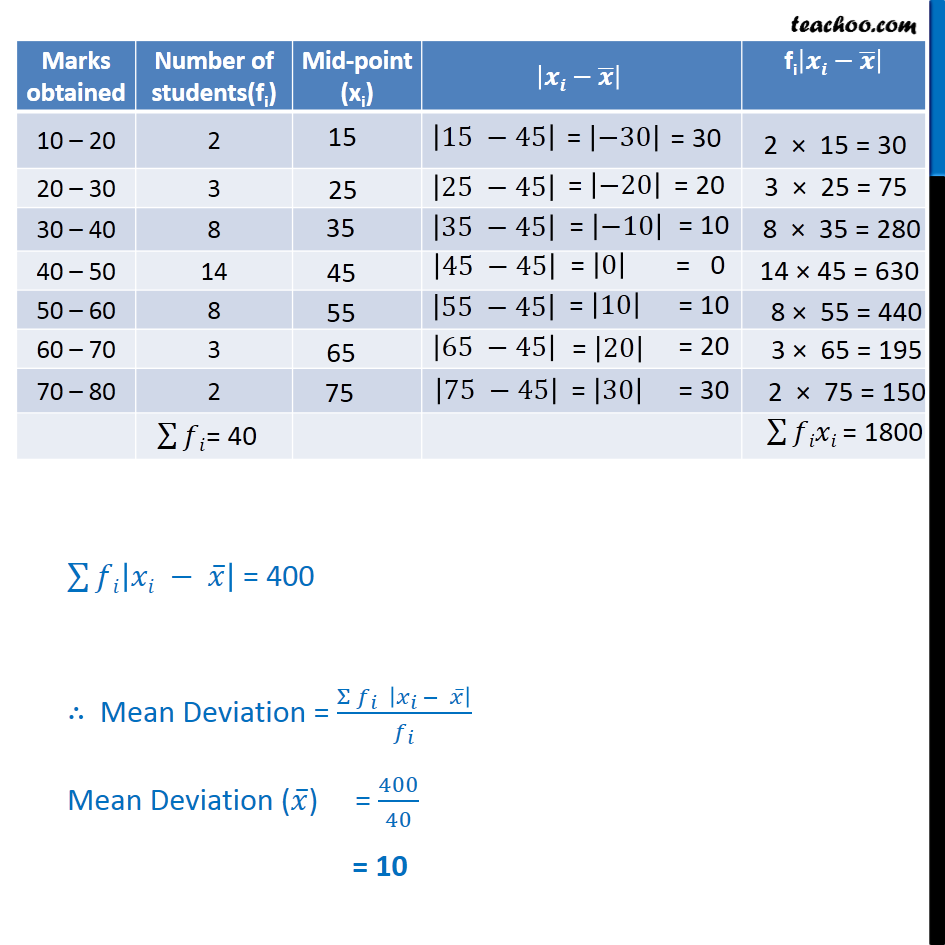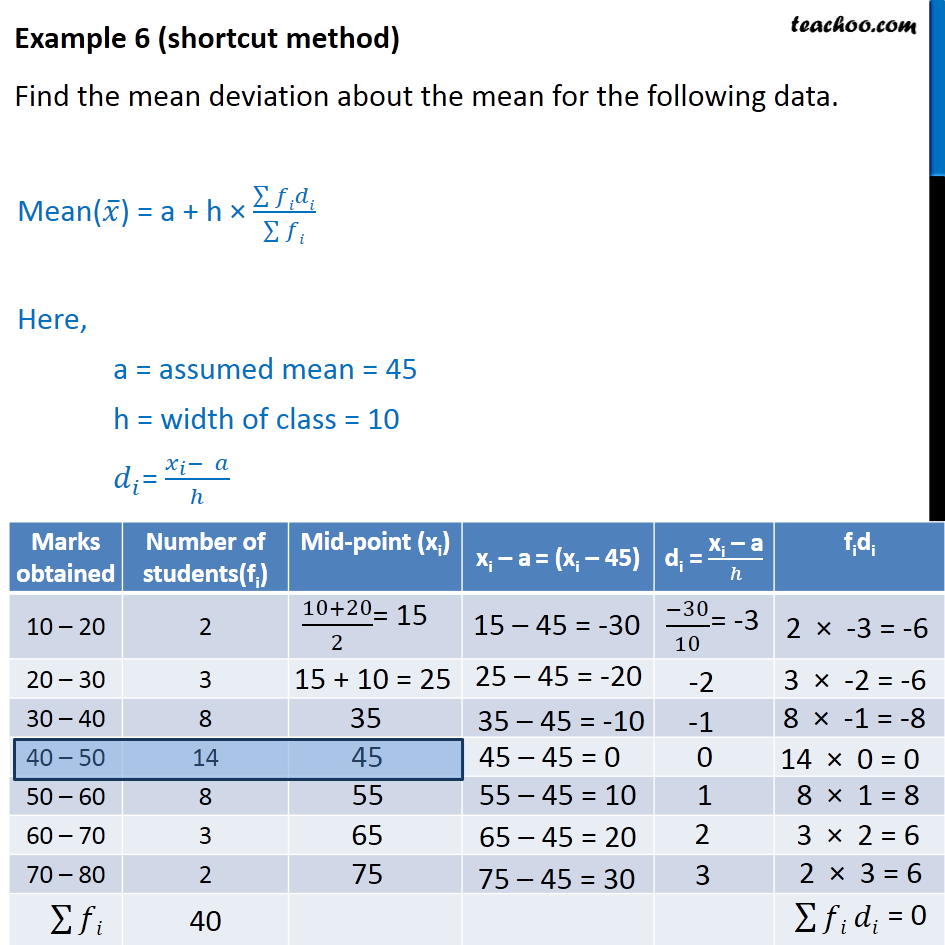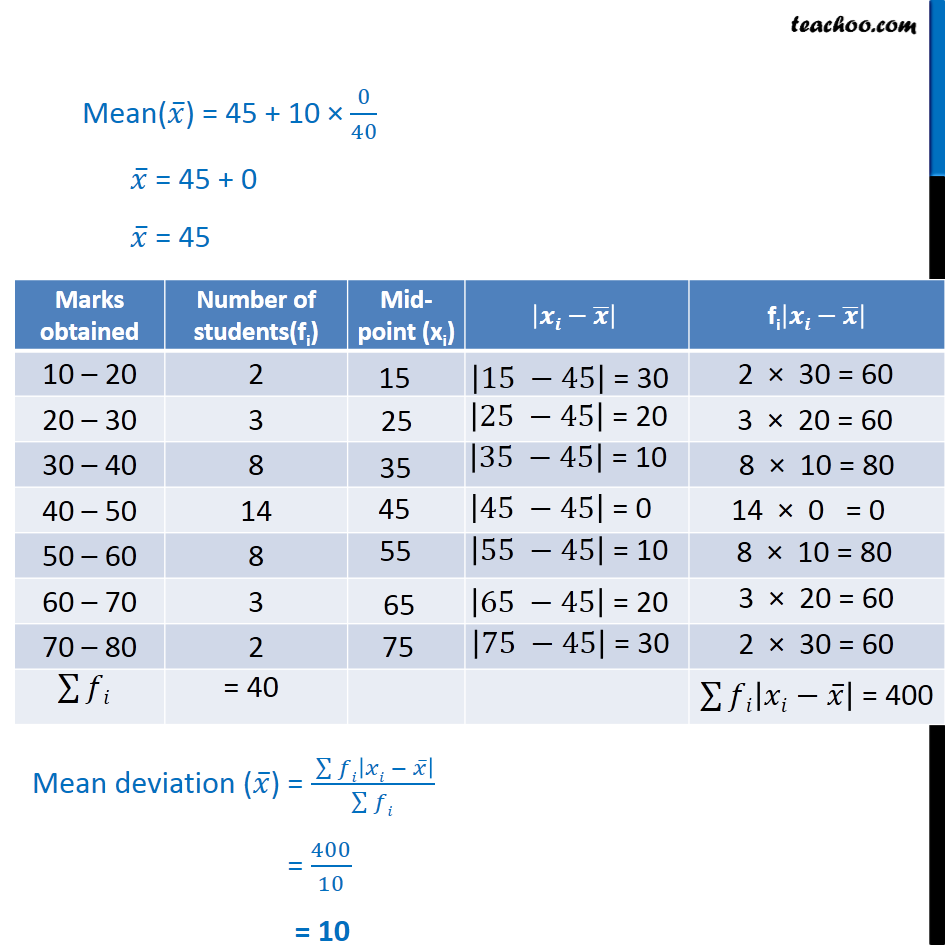Subscribe to our Youtube Channel - https://you.tube/teachoo

1. Chapter 15 Class 11 Statistics
2. Serial order wise
3. Examples

Transcript

Example 6 - Chapter 15 Class 11 Statistics - NCERT (Normal method) Find the mean deviation about the mean for the following data. Marks obtained Number of students(fi) Mid-point (xi) fixi 10 – 20 2 20 – 30 3 30 – 40 8 40 – 50 14 50 – 60 8 60 – 70 3 70 – 80 2 Mean(𝑥 ̅) = (∑▒〖𝑥𝑖 〗 𝑓𝑖)/(∑▒𝑓𝑖) = 1800/40 𝑥 ̅ = 45 ∑128▒𝑓𝑖|𝑥𝑖 − 𝑥 ̅ | = 400 ∴ Mean Deviation = (Σ 𝑓_𝑖 |𝑥_(𝑖 )− 𝑥 ̅ |)/𝑓_𝑖 Mean Deviation (𝑥 ̅) = 400/40 = 10 Example 6 (shortcut method) Find the mean deviation about the mean for the following data. Mean(𝑥 ̅) = a + h × (∑▒𝑓𝑖𝑑𝑖)/(∑▒𝑓𝑖) Here, a = assumed mean = 45 h = width of class = 10 𝑑_𝑖= (𝑥_𝑖− 𝑎)/ℎ Marks obtained Number of students(fi) Mid-point (xi) xi – a = (xi – 45) di = "xi – a" /h fidi 10 – 20 2 20 – 30 3 30 – 40 8 40 – 50 14 50 – 60 8 60 – 70 3 70 – 80 2 40 Marks obtained Number of students(fi) Mid-point (xi) xi – a = (xi – 45) di = "xi – a" /h fidi 10 – 20 2 20 – 30 3 30 – 40 8 40 – 50 14 50 – 60 8 60 – 70 3 70 – 80 2 40 Mean(𝑥 ̅) = 45 + 10 × 0/40 𝑥 ̅ = 45 + 0 𝑥 ̅ = 45 Marks obtained Number of students(fi) Mid-point (xi) |xi-x ̅ | fi|xi-x ̅ | 10 – 20 2 20 – 30 3 30 – 40 8 40 – 50 14 50 – 60 8 60 – 70 3 70 – 80 2 Mean deviation (𝑥 ̅) = ( ∑128▒𝑓𝑖|𝑥𝑖 − 𝑥 ̅ | )/(∑128▒𝑓𝑖) = 400/10 = 10

Examples

Chapter 15 Class 11 Statistics
Serial order wise